## Overview

• Let’s dive a little deeper into candidate generation, aka the first step in our recommendation architecture that we designed above.
• Given a query (user information), the model generates a set of relevant candidates (videos, movies).
• There are two common candidate generation approaches:
1. Content-based filtering: Uses similarity between content to recommend new content.
• For e.g., if user watches corgi videos, the model will recommend more corgi videos.
2. Collaborative filtering: Uses similarity between queries (2 or more users) and items (videos, movies) to provide recommendations.
• For e.g., if user $$A$$ watches corgi videos and user $$A$$ is similar to user $$B$$ (in demographics and other areas), then the model can recommend corgi videos to user $$B$$ even if user $$B$$ has never watched a corgi video before.

## Notation

• A quick note on notation you’ll see in the notes moving forward: we will represent $$r$$ to be ratings, specifically if user $$i$$ has rated item $$j$$, $$n$$ to hold a number value of users or items, and $$y$$ to hold the rating given by a user to a particular item.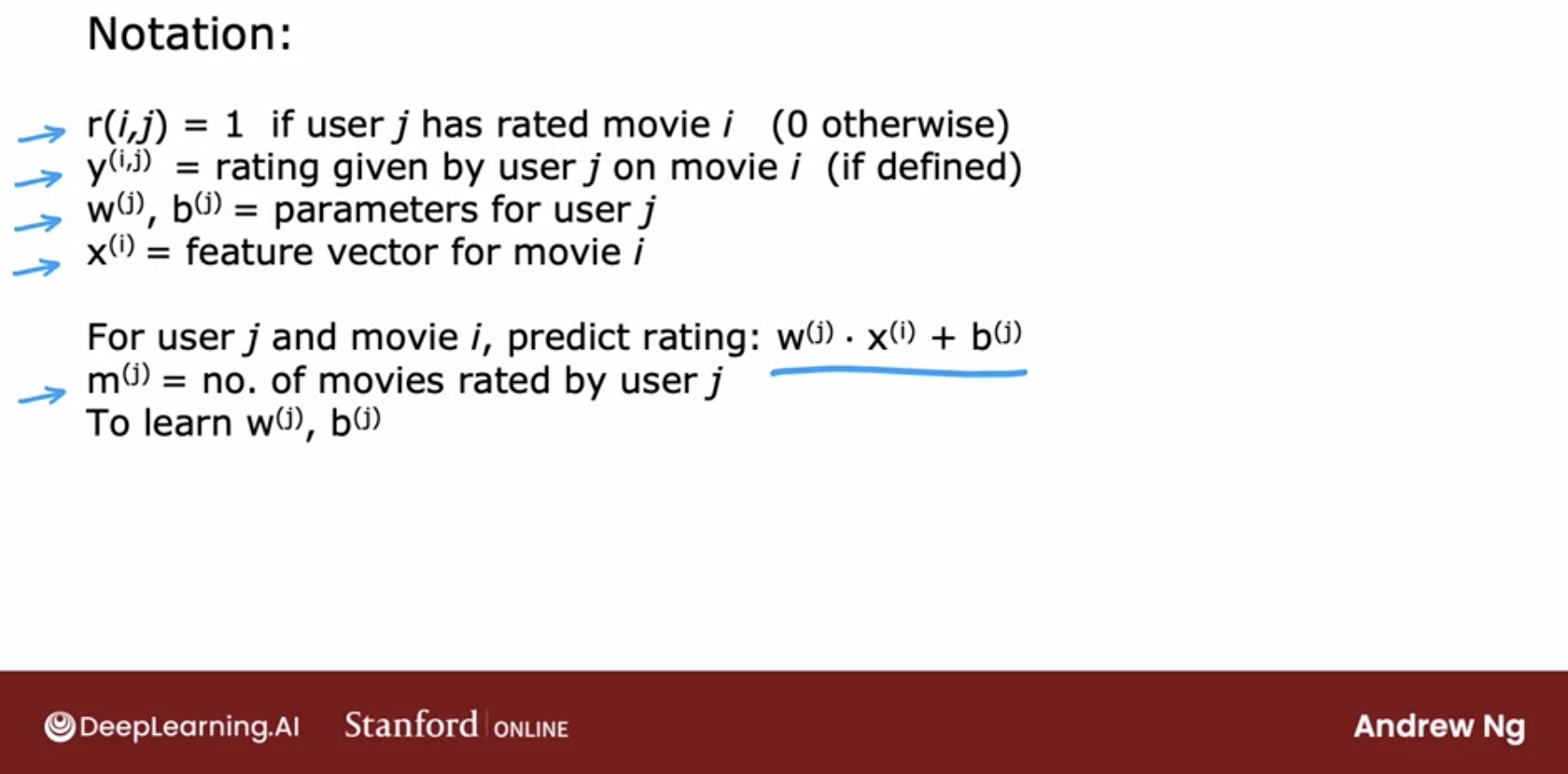## Content-based Filtering

### Overview

• Content-based filtering is when we use documents to recommend other documents that are similar to what the user has liked.

### Pros

• Model does not need data about other users, only needs info for the current user. Makes it easier to scale.
• Can recommend niche items to each user, items that other users may not be interested in.

### Cons

• Requires a lot of domain knowledge as the features are hand-engineered. Thus, the model is only as good as the hand-engineered features.
• Model will only make recommendations based on existing interests of the user and is not able to expand on their interests.

### Example

• Let’s look at an example, first by looking at features: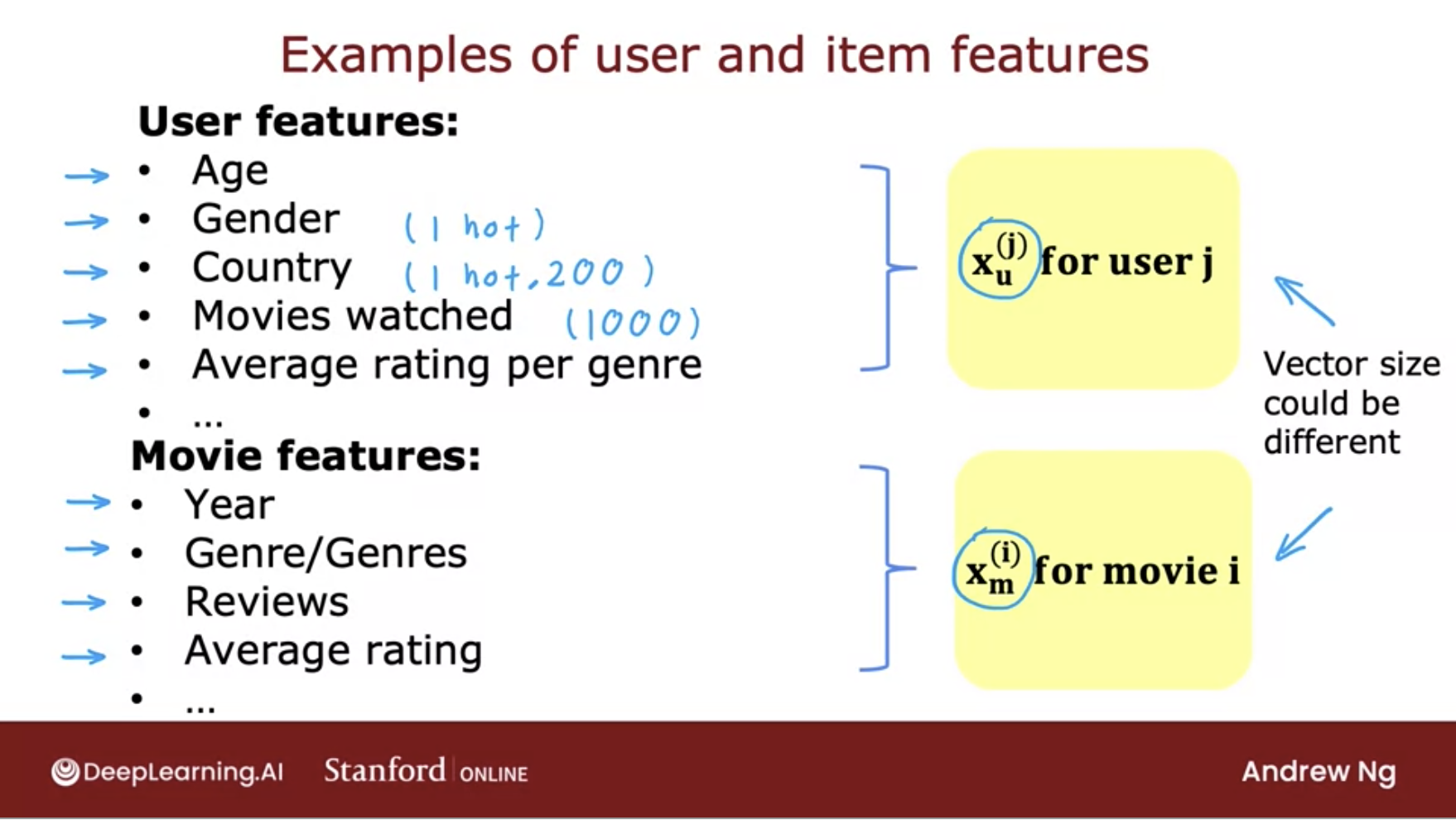• We will be creating an algorithm that learns to match users to items via Deep learning.
• Below is what our architecture will look like: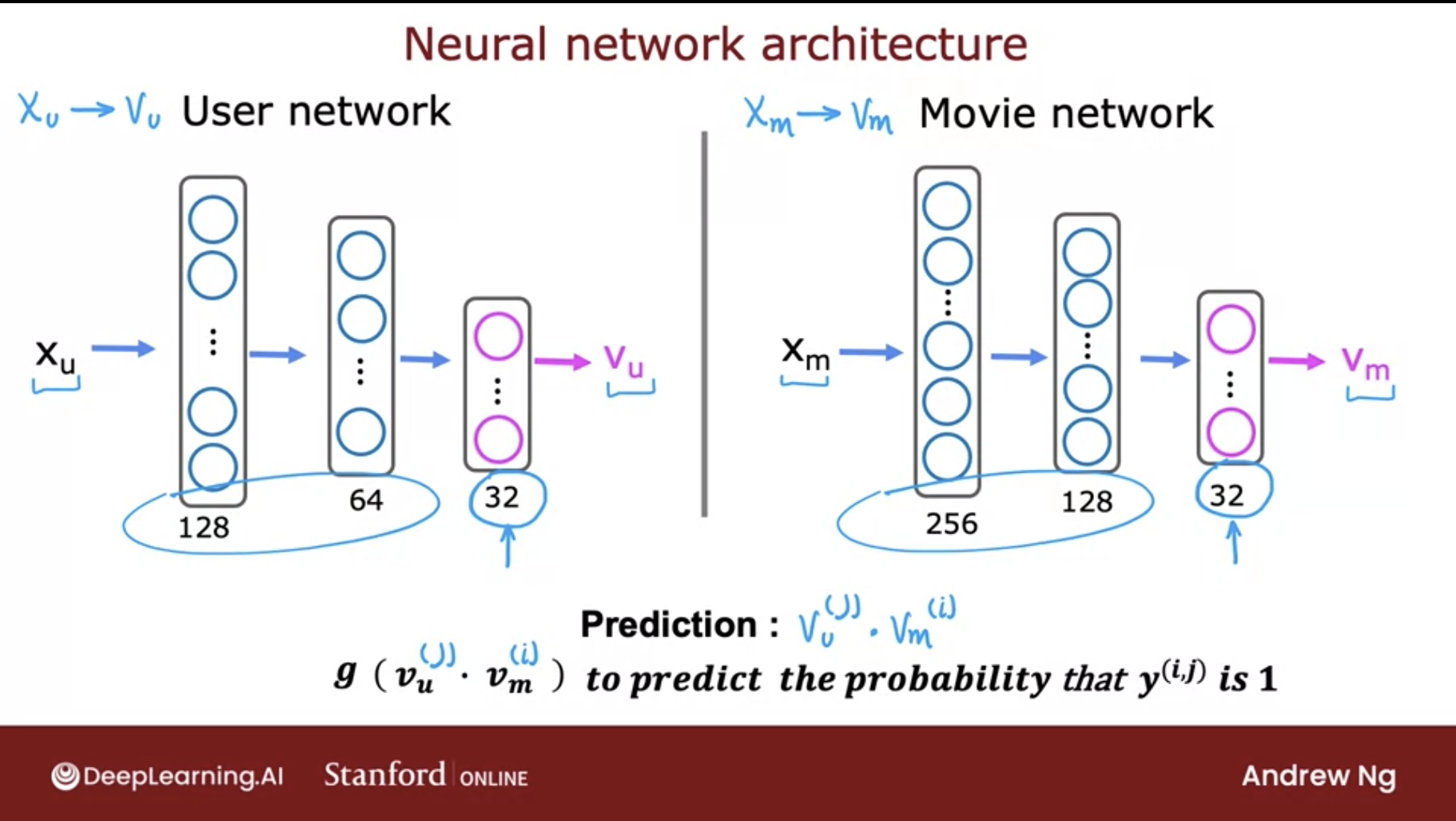• Let’s look at a sequential model in TensorFlow implementing a content-based filtering model: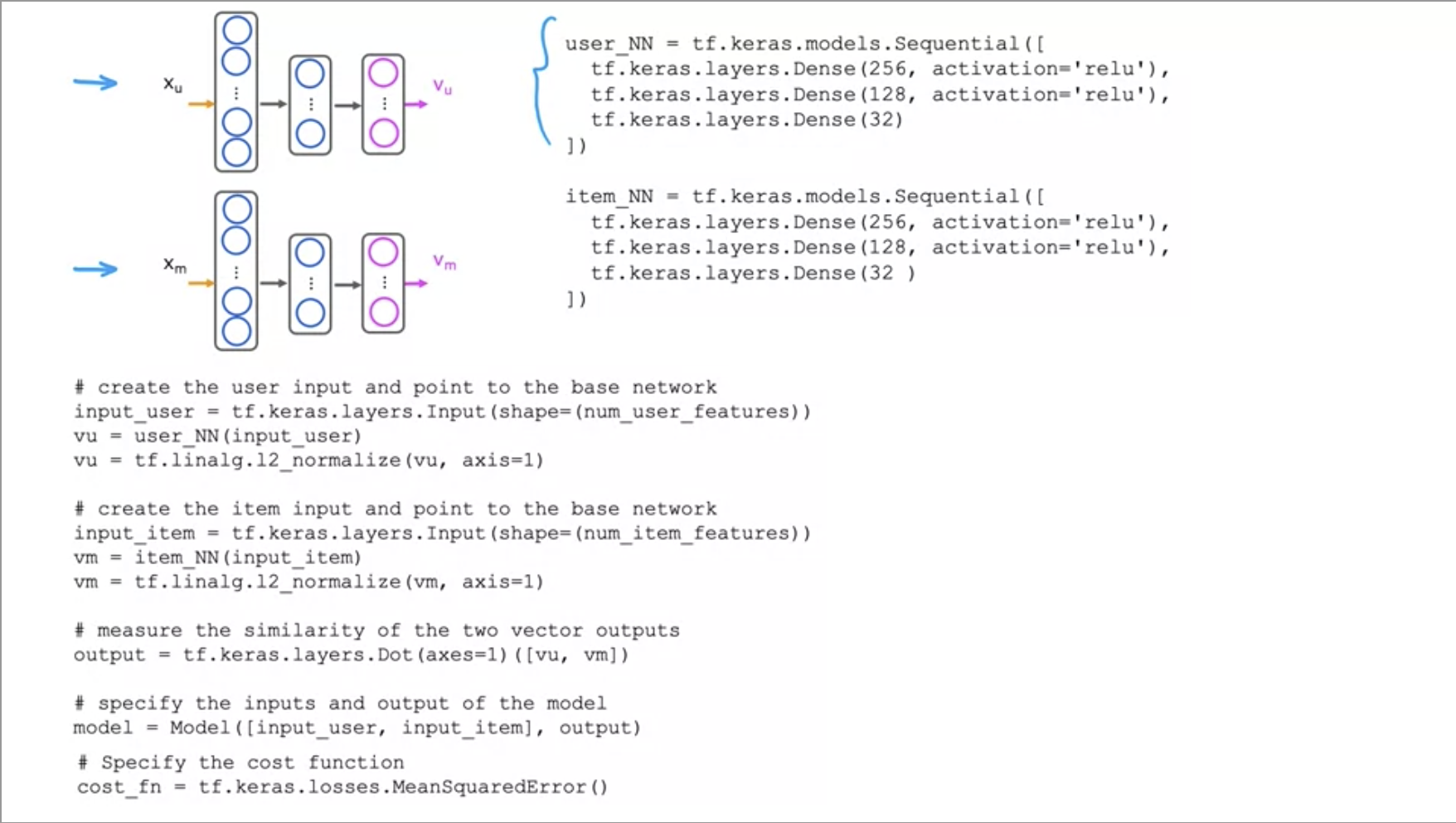• We have 2 dense hidden layers and the final layer outputs 32 numbers. These are all using the ReLu activation function.

### Code deep-dive

• Now, lets build a content-based filtering system using neural networks to recommend movies. The architecture is listed below.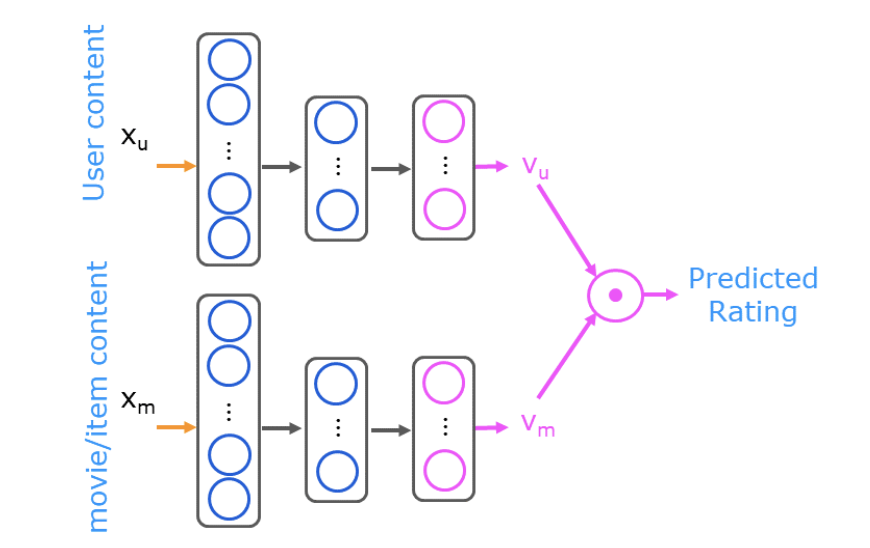• This has been taken from - Coursera: DeepLearning.AI’s specialization
• Lets import our packages and load our dataset:
import numpy as np
import numpy.ma as ma
from numpy import genfromtxt
from collections import defaultdict
import pandas as pd
import tensorflow as tf
from tensorflow import keras
from sklearn.preprocessing import StandardScaler, MinMaxScaler
from sklearn.model_selection import train_test_split
import tabulate
from recsysNN_utils import *
pd.set_option("display.precision", 1)

# Load Data, set configuration variables
item_train, user_train, y_train, item_features, user_features, item_vecs, movie_dict, user_to_genre = load_data()

num_user_features = user_train.shape - 3  # remove userid, rating count and ave rating during training
num_item_features = item_train.shape - 1  # remove movie id at train time
uvs = 3  # user genre vector start
ivs = 3  # item genre vector start
u_s = 3  # start of columns to use in training, user
i_s = 1  # start of columns to use in training, items
scaledata = True  # applies the standard scalar to data if true
print(f"Number of training vectors: {len(item_train)}")

• Let’s take a quick look at our feature vectors and data:
pprint_train(user_train, user_features, uvs,  u_s, maxcount=5)

[user id]	[rating count]	[rating ave]	Act ion	Adve nture	Anim ation	Chil dren	Com edy	Crime	Docum entary	Drama	Fan tasy	Hor ror	Mys tery	Rom ance	Sci -Fi	Thri ller
2	16	4.1	3.9	5.0	0.0	0.0	4.0	4.2	4.0	4.0	0.0	3.0	4.0	0.0	4.2	3.9
2	16	4.1	3.9	5.0	0.0	0.0	4.0	4.2	4.0	4.0	0.0	3.0	4.0	0.0	4.2	3.9
2	16	4.1	3.9	5.0	0.0	0.0	4.0	4.2	4.0	4.0	0.0	3.0	4.0	0.0	4.2	3.9
2	16	4.1	3.9	5.0	0.0	0.0	4.0	4.2	4.0	4.0	0.0	3.0	4.0	0.0	4.2	3.9
2	16	4.1	3.9	5.0	0.0	0.0	4.0	4.2	4.0	4.0	0.0	3.0	4.0	0.0	4.2	3.9


• Let’s prepare the data by doing a bit of preprocessing:
# scale training data
if scaledata:
item_train_save = item_train
user_train_save = user_train

scalerItem = StandardScaler()
scalerItem.fit(item_train)
item_train = scalerItem.transform(item_train)

scalerUser = StandardScaler()
scalerUser.fit(user_train)
user_train = scalerUser.transform(user_train)

print(np.allclose(item_train_save, scalerItem.inverse_transform(item_train)))
print(np.allclose(user_train_save, scalerUser.inverse_transform(user_train)))

• And now split it into test and train:
item_train, item_test = train_test_split(item_train, train_size=0.80, shuffle=True, random_state=1)
user_train, user_test = train_test_split(user_train, train_size=0.80, shuffle=True, random_state=1)
y_train, y_test       = train_test_split(y_train,    train_size=0.80, shuffle=True, random_state=1)
print(f"movie/item training data shape: {item_train.shape}")
print(f"movie/item test  data shape: {item_test.shape}")

movie/item training data shape: (46549, 17)
movie/item test  data shape: (11638, 17)

• Now, let’s construct our neural network as the architecture displayed above. It will have two networks that are combined by a dot product.
# GRADED_CELL
# UNQ_C1

num_outputs = 32
tf.random.set_seed(1)
user_NN = tf.keras.models.Sequential([

tf.keras.layers.Dense(256, activation='relu'),
tf.keras.layers.Dense(128, activation='relu'),
tf.keras.layers.Dense(num_outputs),

])

item_NN = tf.keras.models.Sequential([

tf.keras.layers.Dense(256, activation='relu'),
tf.keras.layers.Dense(128, activation='relu'),
tf.keras.layers.Dense(num_outputs),

])

# create the user input and point to the base network
input_user = tf.keras.layers.Input(shape=(num_user_features))
vu = user_NN(input_user)
vu = tf.linalg.l2_normalize(vu, axis=1)

# create the item input and point to the base network
input_item = tf.keras.layers.Input(shape=(num_item_features))
vm = item_NN(input_item)
vm = tf.linalg.l2_normalize(vm, axis=1)

# compute the dot product of the two vectors vu and vm
output = tf.keras.layers.Dot(axes=1)([vu, vm])

# specify the inputs and output of the model
model = Model([input_user, input_item], output)

model.summary()

Model: "model"
__________________________________________________________________________________________________
Layer (type)                    Output Shape         Param #     Connected to
==================================================================================================
input_1 (InputLayer)            [(None, 14)]         0
__________________________________________________________________________________________________
input_2 (InputLayer)            [(None, 16)]         0
__________________________________________________________________________________________________
sequential (Sequential)         (None, 32)           40864       input_1
__________________________________________________________________________________________________
sequential_1 (Sequential)       (None, 32)           41376       input_2
__________________________________________________________________________________________________
tf_op_layer_l2_normalize/Square [(None, 32)]         0           sequential
__________________________________________________________________________________________________
tf_op_layer_l2_normalize_1/Squa [(None, 32)]         0           sequential_1
__________________________________________________________________________________________________
tf_op_layer_l2_normalize/Sum (T [(None, 1)]          0           tf_op_layer_l2_normalize/Square[0
__________________________________________________________________________________________________
tf_op_layer_l2_normalize_1/Sum  [(None, 1)]          0           tf_op_layer_l2_normalize_1/Square
__________________________________________________________________________________________________
tf_op_layer_l2_normalize/Maximu [(None, 1)]          0           tf_op_layer_l2_normalize/Sum[0
__________________________________________________________________________________________________
tf_op_layer_l2_normalize_1/Maxi [(None, 1)]          0           tf_op_layer_l2_normalize_1/Sum
__________________________________________________________________________________________________
tf_op_layer_l2_normalize/Rsqrt  [(None, 1)]          0           tf_op_layer_l2_normalize/Maximum[
__________________________________________________________________________________________________
tf_op_layer_l2_normalize_1/Rsqr [(None, 1)]          0           tf_op_layer_l2_normalize_1/Maximu
__________________________________________________________________________________________________
tf_op_layer_l2_normalize (Tenso [(None, 32)]         0           sequential
tf_op_layer_l2_normalize/Rsqrt
__________________________________________________________________________________________________
tf_op_layer_l2_normalize_1 (Ten [(None, 32)]         0           sequential_1
tf_op_layer_l2_normalize_1/Rsqrt[
__________________________________________________________________________________________________
dot (Dot)                       (None, 1)            0           tf_op_layer_l2_normalize
tf_op_layer_l2_normalize_1
==================================================================================================
Total params: 82,240
Trainable params: 82,240
Non-trainable params: 0
__________________________________________________________________________________________________

• Finally, it’s time to use a mean squared error loss and an Adam optimizer
tf.random.set_seed(1)
cost_fn = tf.keras.losses.MeanSquaredError()
model.compile(optimizer=opt,
loss=cost_fn)

• Let’s get to training!
tf.random.set_seed(1)
model.fit([user_train[:, u_s:], item_train[:, i_s:]], ynorm_train, epochs=30)

Train on 46549 samples
Epoch 1/30
46549/46549 [==============================] - 6s 122us/sample - loss: 0.1254
Epoch 2/30
46549/46549 [==============================] - 5s 113us/sample - loss: 0.1187
Epoch 3/30
46549/46549 [==============================] - 5s 112us/sample - loss: 0.1169
Epoch 4/30
46549/46549 [==============================] - 5s 118us/sample - loss: 0.1154
Epoch 5/30
46549/46549 [==============================] - 5s 112us/sample - loss: 0.1142
Epoch 6/30
46549/46549 [==============================] - 5s 114us/sample - loss: 0.1130
Epoch 7/30
46549/46549 [==============================] - 5s 114us/sample - loss: 0.1119
Epoch 8/30
46549/46549 [==============================] - 5s 114us/sample - loss: 0.1110
Epoch 9/30
46549/46549 [==============================] - 5s 114us/sample - loss: 0.1095
Epoch 10/30
46549/46549 [==============================] - 5s 113us/sample - loss: 0.1083
Epoch 11/30
46549/46549 [==============================] - 5s 113us/sample - loss: 0.1073
Epoch 12/30
46549/46549 [==============================] - 5s 112us/sample - loss: 0.1066
Epoch 13/30
46549/46549 [==============================] - 5s 113us/sample - loss: 0.1059
Epoch 14/30
46549/46549 [==============================] - 5s 112us/sample - loss: 0.1054
Epoch 15/30
46549/46549 [==============================] - 5s 112us/sample - loss: 0.1047
Epoch 16/30
46549/46549 [==============================] - 5s 114us/sample - loss: 0.1041
Epoch 17/30
46549/46549 [==============================] - 5s 112us/sample - loss: 0.1036
Epoch 18/30
46549/46549 [==============================] - 5s 113us/sample - loss: 0.1030
Epoch 19/30
46549/46549 [==============================] - 5s 112us/sample - loss: 0.1027
Epoch 20/30
46549/46549 [==============================] - 5s 113us/sample - loss: 0.1021
Epoch 21/30
46549/46549 [==============================] - 5s 114us/sample - loss: 0.1018
Epoch 22/30
46549/46549 [==============================] - 5s 112us/sample - loss: 0.1014
Epoch 23/30
46549/46549 [==============================] - 5s 113us/sample - loss: 0.1010
Epoch 24/30
46549/46549 [==============================] - 5s 112us/sample - loss: 0.1006
Epoch 25/30
46549/46549 [==============================] - 5s 116us/sample - loss: 0.1003
Epoch 26/30
46549/46549 [==============================] - 5s 114us/sample - loss: 0.0999
Epoch 27/30
46549/46549 [==============================] - 5s 115us/sample - loss: 0.0997
Epoch 28/30
46549/46549 [==============================] - 5s 112us/sample - loss: 0.0991
Epoch 29/30
46549/46549 [==============================] - 5s 113us/sample - loss: 0.0989
Epoch 30/30
46549/46549 [==============================] - 5s 112us/sample - loss: 0.0985
<tensorflow.python.keras.callbacks.History at 0x7fab691f12d0>

• Evaluate the model to determine the loss on the test data.
model.evaluate([user_test[:, u_s:], item_test[:, i_s:]], ynorm_test)

11638/11638 [==============================] - 0s 33us/sample - loss: 0.1045
0.10449595100221243

• Making predictions is the next step, first lets start off by predicting for a new user:
new_user_id = 5000
new_rating_ave = 1.0
new_action = 1.0
new_animation = 1
new_childrens = 1
new_comedy = 5
new_crime = 1
new_documentary = 1
new_drama = 1
new_fantasy = 1
new_horror = 1
new_mystery = 1
new_romance = 5
new_scifi = 5
new_thriller = 1
new_rating_count = 3

user_vec = np.array([[new_user_id, new_rating_count, new_rating_ave,
new_comedy, new_crime, new_documentary,
new_drama, new_fantasy, new_horror, new_mystery,
new_romance, new_scifi, new_thriller]])

• Let’s look at the top-rated movies for the new user:
# generate and replicate the user vector to match the number movies in the data set.
user_vecs = gen_user_vecs(user_vec,len(item_vecs))

# scale the vectors and make predictions for all movies. Return results sorted by rating.
sorted_index, sorted_ypu, sorted_items, sorted_user = predict_uservec(user_vecs,  item_vecs, model, u_s, i_s,
scaler, scalerUser, scalerItem, scaledata=scaledata)

print_pred_movies(sorted_ypu, sorted_user, sorted_items, movie_dict, maxcount = 10)

y_p	movie id	rating ave	title	genres
4.86762	64969	3.61765	Yes Man (2008)	Comedy
4.86692	69122	3.63158	Hangover, The (2009)	Comedy|Crime
4.86477	63131	3.625	Role Models (2008)	Comedy
4.85853	60756	3.55357	Step Brothers (2008)	Comedy
4.85785	68135	3.55	17 Again (2009)	Comedy|Drama
4.85178	78209	3.55	Get Him to the Greek (2010)	Comedy
4.85138	8622	3.48649	Fahrenheit 9/11 (2004)	Documentary
4.8505	67087	3.52941	I Love You, Man (2009)	Comedy
4.85043	69784	3.65	Brüno (Bruno) (2009)	Comedy
4.84934	89864	3.63158	50/50 (2011)	Comedy|Drama

• Now let’s make predictions for an existing user:
uid =  36
# form a set of user vectors. This is the same vector, transformed and repeated.
user_vecs, y_vecs = get_user_vecs(uid, scalerUser.inverse_transform(user_train), item_vecs, user_to_genre)

# scale the vectors and make predictions for all movies. Return results sorted by rating.
sorted_index, sorted_ypu, sorted_items, sorted_user = predict_uservec(user_vecs, item_vecs, model, u_s, i_s, scaler,
scalerUser, scalerItem, scaledata=scaledata)
sorted_y = y_vecs[sorted_index]

#print sorted predictions
print_existing_user(sorted_ypu, sorted_y.reshape(-1,1), sorted_user, sorted_items, item_features, ivs, uvs, movie_dict, maxcount = 10)

y_p	y	user	user genre ave	movie rating ave	title	genres
3.1	3.0	36	3.00	2.86	Time Machine, The (2002)	Adventure
3.0	3.0	36	3.00	2.86	Time Machine, The (2002)	Action
2.8	3.0	36	3.00	2.86	Time Machine, The (2002)	Sci-Fi
2.3	1.0	36	1.00	4.00	Beautiful Mind, A (2001)	Romance
2.2	1.0	36	1.50	4.00	Beautiful Mind, A (2001)	Drama
1.6	1.5	36	1.75	3.52	Road to Perdition (2002)	Crime
1.6	2.0	36	1.75	3.52	Gangs of New York (2002)	Crime
1.5	1.5	36	1.50	3.52	Road to Perdition (2002)	Drama
1.5	2.0	36	1.50	3.52	Gangs of New York (2002)	Drama



## Collaborative Filtering

### Overview

• To address some of the limitations of content-based filtering, collaborative filtering uses similarities between users and items simultaneously to provide recommendations. This allows for serendipitous recommendations; that is, collaborative filtering models can recommend an item to user $$A$$ based on the interests of a similar user $$B$$.
• In contrast to content-based filtering, collaborative filtering is able to learn embeddings automatically, without relying on hand-engineered features.
• It is also able to take interests of user $$A$$ and recommend it to user $$B$$.
• The goal of a collaborative filtering recommender system is to generate two vectors:
• A ‘parameter vector’ for each user that embodies the item’s tastes of a user.
• A feature vector for each item which embodies some description of the movie.
• The dot product of the two vectors plus the bias term should produce an estimate of the rating the user might give to that movie.

### Pros

• No domain knowledge necessary: We don’t need to have prior domain knowledge as the embeddings are automatically learned.
• Serendipity: The model can help users discover new interests. In isolation, the ML system may not know the user is interested in a given item, but the model might still recommend it because similar users are interested in that item.
• Great starting point: To some extent, the system needs only the feedback matrix to train a matrix factorization model. In particular, the system doesn’t need contextual features. In practice, this can be used as one of multiple candidate generators.

### Cons

• Cold start problem: The prediction of the model for a given (user, item) pair is the dot product of the corresponding embeddings. So, if an item is not seen during training, the system can’t create an embedding for it and can’t query the model with this item. This issue is often called the cold-start problem. Put simply, if the item/document has not been seen during training, our model can not create an embedding from it and can not query the model for this item. However, the following techniques can address the cold-start problem to some extent:
• Projection in WALS: Given a new item $$i_0$$ not seen in training, if the system has a few interactions with users, then the system can easily compute an embedding $$v_{i_0}$$ for this item without having to retrain the whole model. The system simply has to solve the following equation or the weighted version: $$\min _{v_{i_{0}} \in \mathbb{R}^{d}}\left\|A_{i_{0}}-U v_{i_{0}}\right\|$$
• The preceding equation corresponds to one iteration in WALS: the user embeddings are kept fixed, and the system solves for the embedding of item . The same can be done for a new user.
• Heuristics to generate embeddings of fresh items: If the system does not have interactions, the system can approximate its embedding by averaging the embeddings of items from the same category, from the same uploader (in YouTube), and so on.
• Mean Normalization can help with this, as seen in the section on Mean Normalization. Another solution is to show some reasonable items to the new users and have them rate a few items.
• You can also use the user’s information (demographics, expressed preferences, web browser they are using, their IP) to recommend them reasonable items.
• Hard to include side features for query/item: Side features are any features beyond the query or item ID. For movie recommendations, the side features might include country or age. Including available side features improves the quality of the model. Although it may not be easy to include side features in WALS, a generalization of WALS makes this possible.
• To generalize WALS, augment the input matrix with features by defining a block matrix $$\hat{A}$$, where:
• Block (0, 0) is the original feedback matrix $$A$$.
• Block (0, 1) is a multi-hot encoding of the user features.
• Block (1, 0) is a multi-hot encoding of the item features.
• Note: Block (1, 1) is typically left empty. If you apply matrix factorization to , then the system learns embeddings for side features, in addition to user and item embeddings.

### A Movie Recommendation Case-study

• Consider a movie recommendation system (taken from Google’s course on Recommendation Systems) in which the training data consists of a feedback matrix in which:
• Each row represents a user.
• Each column represents an item (a movie).
• The feedback about movies falls into one of two categories:
• Explicit: users specify how much they liked a particular movie by providing a numerical rating.
• Implicit: if a user watches a movie, the system infers that the user is interested.
• To simplify, we will assume that the feedback matrix is binary; that is, a value of 1 indicates interest in the movie.
• When a user visits the homepage, the system should recommend movies based on both:
• Similarity to movies the user has liked in the past.
• Movies that similar users liked.
• For the sake of illustration, let’s hand-engineer some features for the movies described in the following table:#### 1D Embedding

• Suppose we assign to each movie a scalar in [-1, 1] that describes whether the movie is for children (negative values) or adults (positive values). Suppose we also assign a scalar to each user in [-1, 1] that describes the user’s interest in children’s movies (closer to -1) or adult movies (closer to +1). The product of the movie embedding and the user embedding should be higher (closer to 1) for movies that we expect the user to like.• In the diagram below, each checkmark identifies a movie that a particular user watched. The third and fourth users have preferences that are well explained by this feature—the third user prefers movies for children and the fourth user prefers movies for adults. However, the first and second users’ preferences are not well explained by this single feature.#### 2D Embedding

• One feature was not enough to explain the preferences of all users. To overcome this problem, let’s add a second feature: the degree to which each movie is a blockbuster or an arthouse movie. With a second feature, we can now represent each movie with the following two-dimensional embedding:• We again place our users in the same embedding space to best explain the feedback matrix: for each $$(user, item)$$ pair, we would like the dot product of the user embedding and the item embedding to be close to 1 when the user watched the movie, and to 0 otherwise.• Note: We represented both items and users in the same embedding space. This may seem surprising. After all, users and items are two different entities. However, you can think of the embedding space as an abstract representation common to both items and users, in which we can measure similarity or relevance using a similarity metric. In this example, we hand-engineered the embeddings. In practice, the embeddings can be learned automatically, which is the power of collaborative filtering models. In the next two sections, we will discuss different models to learn these embeddings, and how to train them.

• The collaborative nature of this approach is apparent when the model learns the embeddings. Suppose the embedding vectors for the movies are fixed. Then, the model can learn an embedding vector for the users to best explain their preferences. Consequently, embeddings of users with similar preferences will be close together. Similarly, if the embeddings for the users are fixed, then we can learn movie embeddings to best explain the feedback matrix. As a result, embeddings of movies liked by similar users will be close in the embedding space.

#### Examples

• As a real-world example, let’s look at an example with binary labels signifying engagement (using favorites, likes, and clicks):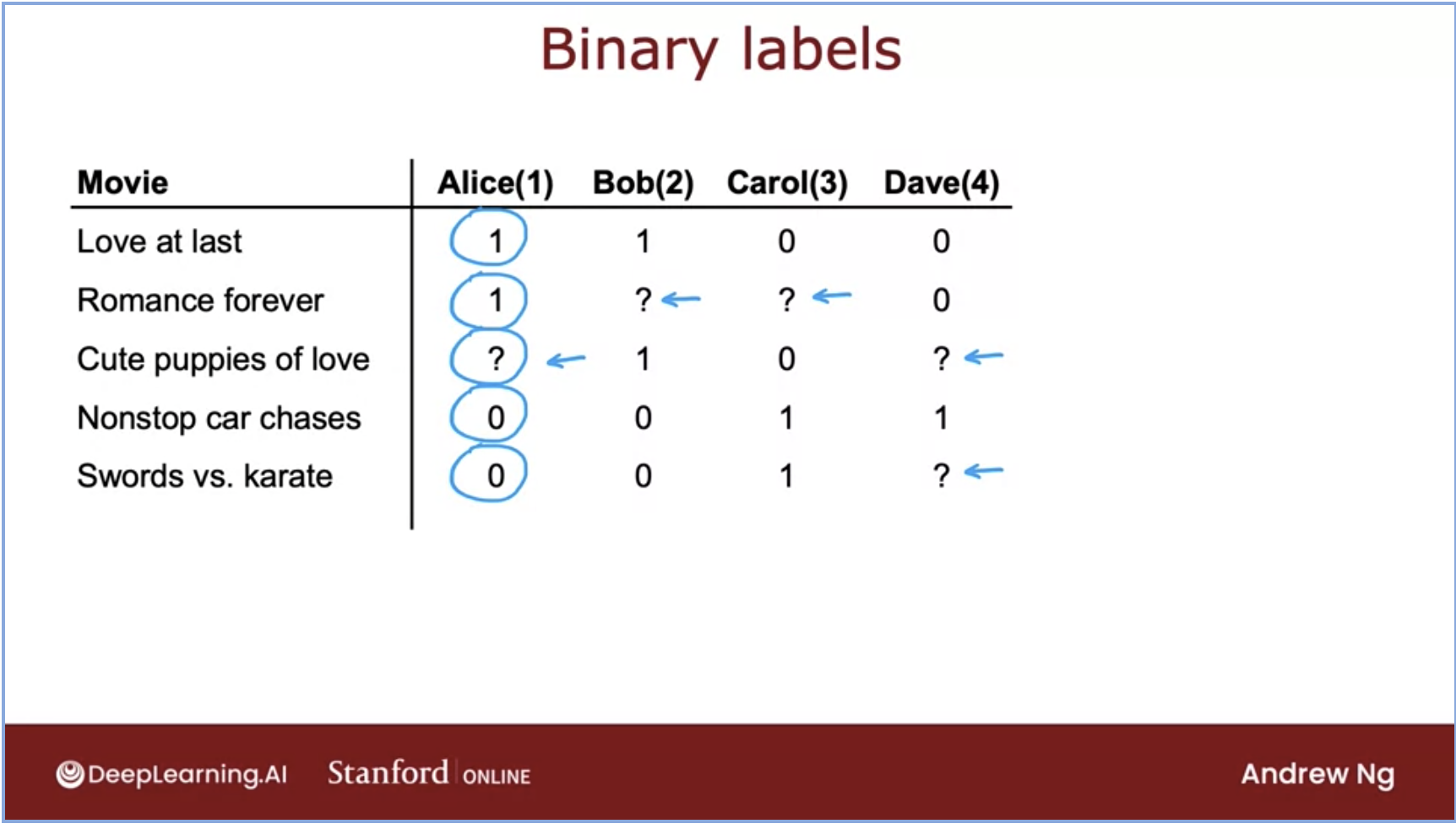• Let’s talk about the meaning of each rating in the above dataset. We can choose what each of our labels mean so here, we have chosen the following:
• 1: user was engaged after being shown the item.
• 0: user did not engage after being shown the item.
• ?: the item is not yet shown to the user.

### Matrix factorization

• Matrix factorization is a simple embedding model. Given the feedback matrix $$\mathrm{A} \in R^{m \times n}$$, where $$m$$ is the number of users (or queries) and $$n$$ is the number of items, the model learns:
• A user embedding matrix $$U \in \mathbb{R}^{m \times d}$$, where row $$i$$ is the embedding for user $$i$$.
• An item embedding matrix $$V \in \mathbb{R}^{n \times d}$$, where row $$j$$ is the embedding for item $$j$$.• The embeddings are learned such that the product $$U V^{T}$$ is a good approximation of the feedback matrix. Observe that the $$(i, j)$$ entry of $$U . V^{T}$$ is simply the dot product $$\left\langle U_{i}, V_{j}\right\rangle$$ of the embeddings of user $$i$$ and item $$j$$, which you want to be close to $$A_{i, j}$$.

• Note: Matrix factorization typically gives a more compact representation than learning the full matrix. The full matrix has $$O(n m)$$ entries, while the embedding matrices $$U, V$$ have $$O((n+m) d)$$ entries, where the embedding dimension $$d$$ is typically much smaller than $$m$$ and $$n$$. As a result, matrix factorization finds latent structure in the data, assuming that observations lie close to a low-dimensional subspace. In the preceding example, the values of $$n, m$$, and $$d$$ are so low that the advantage is negligible. In real-world recommendation systems, however, matrix factorization can be significantly more compact than learning the full matrix.

#### Choosing the Objective Function

• One intuitive objective function is the squared distance. To do this, minimize the sum of squared errors over all pairs of observed entries:
$\min _{U \in \mathbb{R}^{m \times d}, V \in \mathbb{R}^{n \times d}} \sum_{(i, j) \in \mathrm{obs}}\left(A_{i j}-\left\langle U_{i}, V_{j}\right\rangle\right)^{2}$
• In this objective function, you only sum over observed pairs (i, j), that is, over non-zero values in the feedback matrix. However, only summing over values of one is not a good idea-a matrix of all ones will have a minimal loss and produce a model that can’t make effective recommendations and that generalizes poorly.• Perhaps you could treat the unobserved values as zero, and sum over all entries in the matrix. This corresponds to minimizing the squared Frobenius distance between $$A$$ and its approximation $$U V^{T}$$:
$\min _{U \in \mathbb{R}^{m \times d}, V \in \mathbb{R}^{n \times d}}\left\|A-U V^{T}\right\|_{F}^{2} .$
• You can solve this quadratic problem through Singular Value Decomposition (SVD) of the matrix. However, SVD is not a great solution either, because in real applications, the matrix $$A$$ may be very sparse. For example, think of all the videos on YouTube compared to all the videos a particular user has viewed. The solution $$U V^{T}$$ (which corresponds to the model’s approximation of the input matrix) will likely be close to zero, leading to poor generalization performance.

• In contrast, Weighted Matrix Factorization decomposes the objective into the following two sums:

• A sum over observed entries.
• A sum over unobserved entries (treated as zeroes).
$\min _{U \in \mathbb{R}^{m \times d}, V \in \mathbb{R}^{n \times d}} \sum_{(i, j) \in \mathrm{obs}}\left(A_{i j}-\left\langle U_{i}, V_{j}\right\rangle\right)^{2}+w_{0} \sum_{(i, j) \notin \mathrm{obs}}\left(\left\langle U_{i}, V_{j}\right\rangle\right)^{2}$
• Here, $$w_{0}$$ is a hyperparameter that weights the two terms so that the objective is not dominated by one or the other. Tuning this hyperparameter is very important.

• Note: In practical applications, you also need to weight the observed pairs carefully. For example, frequent items (for example, extremely popular YouTube videos) or frequent queries (for example, heavy users) may dominate the objective function. You can correct for this effect by weighting training examples to account for item frequency. In other words, you can replace the objective function by:

$\sum_{(i, j) \in \mathrm{obs}} w_{i, j}\left(A_{i, j}-\left\langle U_{i}, V_{j}\right\rangle\right)^{2}+w_{0} \sum_{i, j \notin \mathrm{obs}}\left\langle U_{i}, V_{j}\right\rangle^{2}$
• where $$w_{i, j}$$ is a function of the frequency of query $$\mathrm{i}$$ and item $$\mathrm{j}$$.

### Minimizing the Objective Function

• Common algorithms to minimize the objective function include:
• Stochastic gradient descent (SGD) is a generic method to minimize loss functions.
• Weighted Alternating Least Squares (WALS) is specialized to this particular objective. The objective is quadratic in each of the two matrices $$\mathrm{U}$$ and $$\mathrm{V}$$. (Note, however, that the problem is not jointly convex.) WALS works by initializing the embeddings randomly, then alternating between:
• Fixing $$U$$ and solving for $$V$$.
• Fixing $$V$$ and solving for $$U$$.
• Each stage can be solved exactly (via solution of a linear system) and can be distributed. This technique is guaranteed to converge because each step is guaranteed to decrease the loss.

#### Cost function for binary labels (regression to classification)

• Let’s look at how we can generalize our algorithm, note that this is a lot like linear regression but we instead to predict these binary outputs, thus move from regression to a binary classification problem.
• Below we have the cost function for this binary application: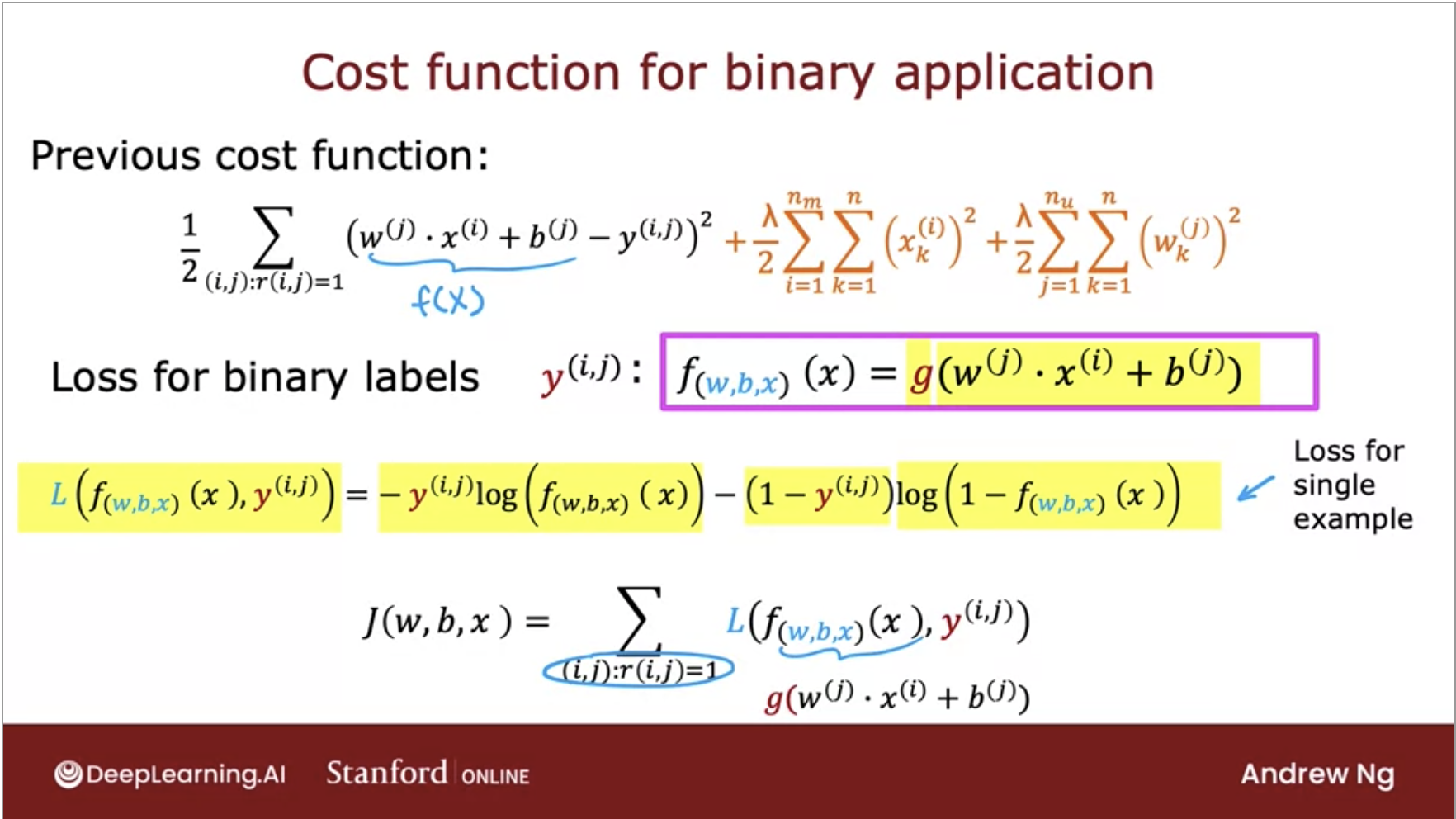• This is how we take linear regression-like collaborative filtering algorithm and generalize it to work for binary labels.

### SGD vs. WALS

• SGD and WALS have advantages and disadvantages. Review the information below to see how they compare:

#### SGD

• (+) Very flexible—can use other loss functions.
• (+) Can be parallelized.
• (-) Slower—does not converge as quickly.
• (-) Harder to handle the unobserved entries (need to use negative sampling or gravity).

#### WALS

• (-) Reliant on Loss Squares only.
• (+) Can be parallelized.
• (+) Converges faster than SGD.
• (+) Easier to handle unobserved entries.

### Example: Learning process for vectors

• Below is a diagram of how vectors are learned using matrix factorization.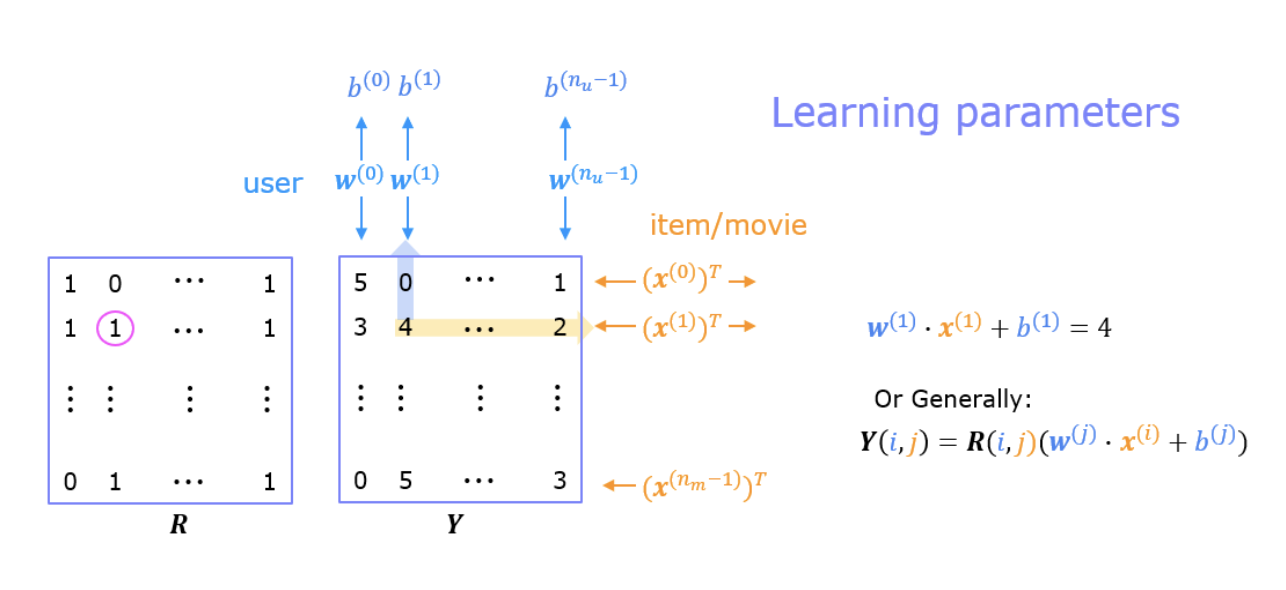• $$Y$$ contains ratings; 0.5 to 5 inclusive in 0.5 steps. 0 if the movie has not been rated.
• $$R$$ has a 1 where movies have been rated.
• Movies are in rows, users in columns. Each user has a parameter vector $$w_{user}$$ and bias. Each movie has a feature vector $$x_{movie}$$. These vectors are simultaneously learned by using the existing user/movie ratings as training data.
• One training example is shown above: $$w^{(1)} \cdot x^{(1)} + b^{(1)}=4$$.
• The feature vector $$x_{movie}$$ must satisfy all the users while the user vector $$w_{user}$$ must satisfy all the movies. Since all the users collaborate to generate the rating set, we call it collaborative filtering.
• Once the feature vectors and parameters are learned, they can be used to predict how a user might rate an unrated movie.

### Code deep-dive

• Now lets look into the code for a movie recommendation model from Andrew Ng’s Coursera specialization.
• The first part of the exercise was to implement the collaborative filtering cost function. Let’s skip ahead to training:
    movieList, movieList_df = load_Movie_List_pd()

my_ratings = np.zeros(num_movies) #  Initialize my ratings

# Check the file small_movie_list.csv for id of each movie in our dataset
# For example, Toy Story 3 (2010) has ID 2700, so to rate it "5", you can set
my_ratings = 5

#Or suppose you did not enjoy Persuasion (2007), you can set
my_ratings = 2;

# We have selected a few movies we liked / did not like and the ratings we gave are as follows:
my_ratings  = 5   # Lord of the Rings: The Return of the King, The
my_ratings  = 5   # Shrek (2001)
my_ratings = 3   # Inception
my_ratings = 5   # Incredibles, The (2004)
my_ratings  = 2   # Amelie (Fabuleux destin d'Amélie Poulain, Le)
my_ratings  = 5   # Harry Potter and the Sorcerer's Stone (a.k.a. Harry Potter and the Philosopher's Stone) (2001)
my_ratings  = 5   # Harry Potter and the Chamber of Secrets (2002)
my_ratings  = 3   # Eternal Sunshine of the Spotless Mind (2004)
my_ratings = 1   # Louis Theroux: Law & Disorder (2008)
my_ratings = 1   # Nothing to Declare (Rien à déclarer)
my_ratings  = 5   # Pirates of the Caribbean: The Curse of the Black Pearl (2003)
my_rated = [i for i in range(len(my_ratings)) if my_ratings[i] > 0]

print('\nNew user ratings:\n')
for i in range(len(my_ratings)):
if my_ratings[i] > 0 :
print(f'Rated {my_ratings[i]} for  {movieList_df.loc[i,"title"]}');

    New user ratings:

Rated 5.0 for  Shrek (2001)
Rated 5.0 for  Harry Potter and the Sorcerer's Stone (a.k.a. Harry Potter and the Philosopher's Stone) (2001)
Rated 2.0 for  Amelie (Fabuleux destin d'Amélie Poulain, Le) (2001)
Rated 5.0 for  Harry Potter and the Chamber of Secrets (2002)
Rated 5.0 for  Pirates of the Caribbean: The Curse of the Black Pearl (2003)
Rated 5.0 for  Lord of the Rings: The Return of the King, The (2003)
Rated 3.0 for  Eternal Sunshine of the Spotless Mind (2004)
Rated 5.0 for  Incredibles, The (2004)
Rated 2.0 for  Persuasion (2007)
Rated 5.0 for  Toy Story 3 (2010)
Rated 3.0 for  Inception (2010)
Rated 1.0 for  Louis Theroux: Law & Disorder (2008)
Rated 1.0 for  Nothing to Declare (Rien à déclarer) (2010)

• Above we are starting with my ratings and printing all the ratings > 0.
• Now let’s add these reviews to 𝑌 and 𝑅 and normalize the ratings.
  # Reload ratings and add new ratings
Y    = np.c_[my_ratings, Y]
R    = np.c_[(my_ratings != 0).astype(int), R]

# Normalize the Dataset
Ynorm, Ymean = normalizeRatings(Y, R)

• Now, let’s initialize the parameters and select the Adam optimizer
  #  Useful Values
num_movies, num_users = Y.shape
num_features = 100

# Set Initial Parameters (W, X), use tf.Variable to track these variables
tf.random.set_seed(1234) # for consistent results
W = tf.Variable(tf.random.normal((num_users,  num_features),dtype=tf.float64),  name='W')
X = tf.Variable(tf.random.normal((num_movies, num_features),dtype=tf.float64),  name='X')
b = tf.Variable(tf.random.normal((1,          num_users),   dtype=tf.float64),  name='b')

# Instantiate an optimizer.

• The final step left here is to train our collaborative filtering model. This will learn the parameters $$X$$, $$W$$, and $$b$$.
• Since learning these parameters doesn’t follow the typical ‘layers’ offered in TensorFlow neural network package, we will need to use custom training loop instead of the traditional model flow: compile(), fit(), and predict().
• In order to do this,we will first return the gradient of the loss relative to the tracked variables.
• Further, the gradients can then be applied to the parameters using an optimizer.
  iterations = 200
lambda_ = 1
for iter in range(iterations):
# to record the operations used to compute the cost

# Compute the cost (forward pass included in cost)
cost_value = cofi_cost_func_v(X, W, b, Ynorm, R, lambda_)

# Use the gradient tape to automatically retrieve
# the gradients of the trainable variables with respect to the loss

# Run one step of gradient descent by updating
# the value of the variables to minimize the loss.

# Log periodically.
if iter % 20 == 0:
print(f"Training loss at iteration {iter}: {cost_value:0.1f}")

• Below is the loss we had logged periodically:
  Training loss at iteration 0: 2321138.6
Training loss at iteration 20: 136158.3
Training loss at iteration 40: 51858.7
Training loss at iteration 60: 24596.9
Training loss at iteration 80: 13629.5
Training loss at iteration 100: 8487.1
Training loss at iteration 120: 5807.2
Training loss at iteration 140: 4311.2
Training loss at iteration 160: 3434.9
Training loss at iteration 180: 2901.7

• Below we will start making our predictions which will be based off our initial ratings given earlier. We can compare the original and predicted value in the output below:
  # Make a prediction using trained weights and biases
p = np.matmul(X.numpy(), np.transpose(W.numpy())) + b.numpy()

#restore the mean
pm = p + Ymean

my_predictions = pm[:,0]

# sort predictions
ix = tf.argsort(my_predictions, direction='DESCENDING')

for i in range(17):
j = ix[i]
if j not in my_rated:
print(f'Predicting rating {my_predictions[j]:0.2f} for movie {movieList[j]}')

print('\n\nOriginal vs Predicted ratings:\n')
for i in range(len(my_ratings)):
if my_ratings[i] > 0:
print(f'Original {my_ratings[i]}, Predicted {my_predictions[i]:0.2f} for {movieList[i]}')

  Predicting rating 4.55 for movie My Sassy Girl (Yeopgijeogin geunyeo) (2001)
Predicting rating 4.53 for movie Martin Lawrence Live: Runteldat (2002)
Predicting rating 4.53 for movie Delirium (2014)
Predicting rating 4.53 for movie Particle Fever (2013)
Predicting rating 4.53 for movie Laggies (2014)
Predicting rating 4.53 for movie One I Love, The (2014)
Predicting rating 4.52 for movie Eichmann (2007)
Predicting rating 4.52 for movie Battle Royale 2: Requiem (Batoru rowaiaru II: Chinkonka) (2003)
Predicting rating 4.52 for movie Into the Abyss (2011)
Predicting rating 4.52 for movie Son of the Bride (Hijo de la novia, El) (2001)
Predicting rating 4.51 for movie Rivers and Tides (2001)

Original vs Predicted ratings:

Original 5.0, Predicted 4.89 for Shrek (2001)
Original 5.0, Predicted 4.84 for Harry Potter and the Sorcerer's Stone (a.k.a. Harry Potter and the Philosopher's Stone) (2001)
Original 2.0, Predicted 2.13 for Amelie (Fabuleux destin d'Amélie Poulain, Le) (2001)
Original 5.0, Predicted 4.89 for Harry Potter and the Chamber of Secrets (2002)
Original 5.0, Predicted 4.91 for Lord of the Rings: The Return of the King, The (2003)
Original 3.0, Predicted 3.00 for Eternal Sunshine of the Spotless Mind (2004)
Original 5.0, Predicted 4.89 for Incredibles, The (2004)
Original 2.0, Predicted 2.15 for Persuasion (2007)
Original 5.0, Predicted 4.80 for Toy Story 3 (2010)
Original 3.0, Predicted 3.01 for Inception (2010)
Original 1.0, Predicted 1.48 for Louis Theroux: Law & Disorder (2008)


• Below we have a dataframe showing data from the top 20 ratings:
  filter=(movieList_df["number of ratings"] > 20)
movieList_df["pred"] = my_predictions
movieList_df = movieList_df.reindex(columns=["pred", "mean rating", "number of ratings", "title"])
movieList_df.loc[ix[:300]].loc[filter].sort_values("mean rating", ascending=False)

  pred  mean rating number of ratings title
2112  4.097693  4.238255  149 Dark Knight, The (2008)
155 4.004862  4.155914  93  Snatch (2000)
211 4.246676  4.122642  159 Memento (2000)
929 4.911655  4.118919  185 Lord of the Rings: The Return of the King, The...
2700  4.795389  4.109091  55  Toy Story 3 (2010)
653 4.331339  4.021277  188 Lord of the Rings: The Two Towers, The (2002)
2804  4.393629  3.989362  47  Harry Potter and the Deathly Hallows: Part 1 (...
773 4.082829  3.960993  141 Finding Nemo (2003)
1771  4.246275  3.944444  81  Casino Royale (2006)
2455  4.244220  3.887931  58  Harry Potter and the Half-Blood Prince (2009)
361 4.052389  3.871212  132 Monsters, Inc. (2001)
246 4.893447  3.867647  170 Shrek (2001)
1150  4.885246  3.836000  125 Incredibles, The (2004)
366 4.839989  3.761682  107 Harry Potter and the Sorcerer's Stone (a.k.a. ...
79  4.226652  3.699248  133 X-Men (2000)
622 4.889378  3.598039  102 Harry Potter and the Chamber of Secrets (2002)


## Retrieval

• Suppose you have an embedding model. Given a user, how would you decide which items to recommend? At serve time, given a query, you start by doing one of the following:
• For a matrix factorization model, the query (or user) embedding is known statically, and the system can simply look it up from the user embedding matrix.
• For a DNN model, the system computes the query embedding $$\psi(x)$$ at serve time by running the network on the feature vector $$x$$.
• Once you have the query embedding $q$, search for item embeddings $$V_{j}$$ that are close to $$q$$ in the embedding space. This is a nearest neighbor problem. For example, you can return the top $$k$$ items according to the similarity score $$s\left(q, V_{j}\right)$$.• You can use a similar approach in related-item recommendations. For example, when the user is watching a YouTube video, the system can first look up the embedding of that item, and then look for embeddings of other items that are close in the embedding space.

### Large-scale Retrieval

• To compute the nearest neighbors in the embedding space, the system can exhaustively score every potential candidate. Exhaustive scoring can be expensive for very large corpora, but you can use either of the following strategies to make it more efficient:
• If the query embedding is known statically, the system can perform exhaustive scoring offline, precomputing and storing a list of the top candidates for each query. This is a common practice for related-item recommendation.
• Use approximate nearest neighbors.